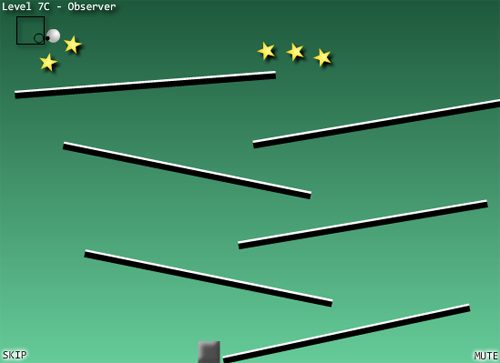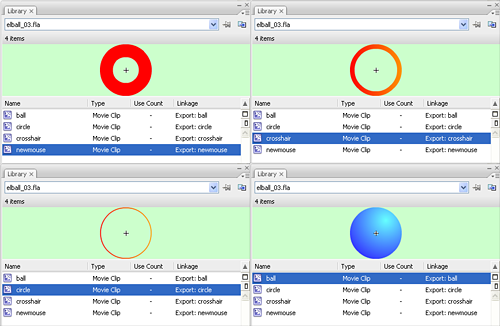# Controlling a ball like in Flash Elasticity game tutorial

Due to the upcoming deadline of the Flash Game Design Competition with “Ball Phyiscs” theme, here it is another ball physics tutorial.

I was looking for some good ball physics concepts and I stumbled upon Elasticity, a nice game with an interesting way to controll the ball.Why don’t we try to control a ball like in this game? Let’s try to understand how does it work: There is a dot that you move with the mouse, a black circle that tries to follow the dot, a black square that represents the movement limit of the black circle, and a ball that follows the black circle with an elastic effect (yes, play it some minutes before reading this tutorial because I realize the description sucks).

We are going to create a control system like this one in less than 40 lines, brackets and declarations included.

Before we start, let me introduce the movieclips with their linksball: it’s the ball itself

circle: it’s the circle that limits movements. In Elasticity the authot used a square, but with a circle we are forced to use a bit of trigonometry that is always a good thing to do

crosshair: the crosshair that follows the mouse, limited by the circle

newmouse: the clip that will replace current mouse pointer

Now, as usual, we have all the actionscript in the first frame:

```attachMovie("newmouse","newmouse",_root.getNextHighestDepth());
attachMovie("circle","circle",_root.getNextHighestDepth(),{_x:250, _y:200});
attachMovie("crosshair","crosshair",_root.getNextHighestDepth());
attachMovie("ball","ball",_root.getNextHighestDepth());
Mouse.hide();
friction = 0.9;
speed_scale = 0.1;
xspeed = 0;
yspeed = 0;
newmouse.onEnterFrame = function() {
this._x = _root._xmouse;
this._y = _root._ymouse;
};
crosshair.onEnterFrame = function() {
this._x = _root._xmouse;
this._y = _root._ymouse;
dist_x = this._x-circle._x;
dist_y = this._y-circle._y;
distance = Math.sqrt(dist_x*dist_x+dist_y*dist_y);
if (distance>45) {
angle = Math.atan2(dist_y, dist_x);
this._x = 250+45*Math.cos(angle);
this._y = 200+45*Math.sin(angle);
}

};
ball.onEnterFrame = function() {
dist_x = (crosshair._x-this._x)*speed_scale;
dist_y = (crosshair._y-this._y)*speed_scale;
xspeed += dist_x;
yspeed += dist_y;
xspeed *= friction;
yspeed *= friction;
this._x += xspeed;
this._y += yspeed;
};```

Just 36 lines of easy code. Let’s see them:

Lines 1-4: Attaching the movieclips to stage. Notice that they haven’t an initial position, with the only exception of the circle that in this case I am placing in the centre of the stage.

Lines 5: Hiding the mouse pointer

Line 6: Defining the friction. I discussed about friction in this tutorial

Line 7: This is a pretty useless variable, but I had to use it. I’ll show you how does it work later

Lines 8-9: Defining x and y speeds. These values refer to ball speed

Line 10: Beginning of the routine to be executed at every frame for the newmouse object

Lines 11-12: Simply adjusting newmouse _x and _y positions according to the mouse position. In other words, the player will use newmouse as a new mouse pointer

Line 14: Beginning of the routine to be executed at every frame for the crosshair object

Lines 15-16: Placing this movieclip in the same mouse position as seen for newmouse. Both movieclips have to follow the mouse. The “only” difference is that while the newmouse always follows the mouse, the crosshair follows the mouse only when it’s inside the circle.

Lines 17-19: Calculating the distance between the centre of the circle and the centre of the crosshair with the Pythagorean theorem

Line 20: Controlling if the distance is greater than 45 pixels. In this case, it means the crosshair is outside the circle. Why “45”? Because it’s the circle radius (50) minus the crosshair radius (5). I don’t want the crosshair to go outside the circle, but I want it to place as near as it can to the mouse, just remaining inside the circle.

Line 21: Determining the angle between the centre of the circle and the centre of the crosshair using trigonometry. Some basics of Flash trigonometry are explained in this tutorial.

Lines 22-23: Placing the crosshair in the same direction of the mouse pointer, just inside the circle, using trigonometry. Notice that you can write `circle._x` and `circle._y` instead of 250 and 200 respectively.

Line 27: Beginning of the routine to be executed at every frame for the ball object, the last one.

Lines 28-29: Finding the x and y distance between the crosshair (look: the crosshair, not the mouse) and the ball. Here is where I use speed_scale variable: I just found the ball going too fast and I had to scale down speed, so now I divide the x and y distance by 10 (or multiply by 0.1, it’s the same isn’t it?) to have the game more playable.

Lines 30-31: Adding the x and y distances to current x and y speeds (this simulates the “elastic”… the greater the distance between the ball and the crosshair, the greater the speed of the ball in crosshair direction)

Lines 32-33: Applying the friction

Lines 34-35: Updating ball position

That’s it! Less than 40 lines as you can see. Now it’s up to you to decide what can you do with this ball… I am planning to break some bricks, time to play a bit with actionscript, meanwhile take the source code.

214 GAME PROTOTYPES EXPLAINED WITH SOURCE CODE
// 1+2=3
// 10000000
// 2 Cars
// 2048
// Avoider
// Ballz
// Block it
// Blockage
// Bloons
// Boids
// Bombuzal
// Breakout
// Bricks
// Columns
// CubesOut
// Dots
// DROP'd
// Dudeski
// Eskiv
// Filler
// Fling
// Globe
// HookPod
// Hundreds
// InkTd
// Iromeku
// Lumines
// Magick
// MagOrMin
// Maze
// Memdot
// Nano War
// Nodes
// o:anquan
// Ononmin
// Pacco
// Phyballs
// Platform
// Poker
// Pool
// Poux
// Pudi
// qomp
// Racing
// Renju
// SameGame
// Security
// Sling
// Slingy
// Sokoban
// Splitter
// Sproing
// Stack
// Stringy
// Sudoku
// Tetris
// Threes
// Toony
// Turn
// TwinSpin
// vvvvvv
// Wordle
// Worms
// Yanga
// Zhed
// zNumbers